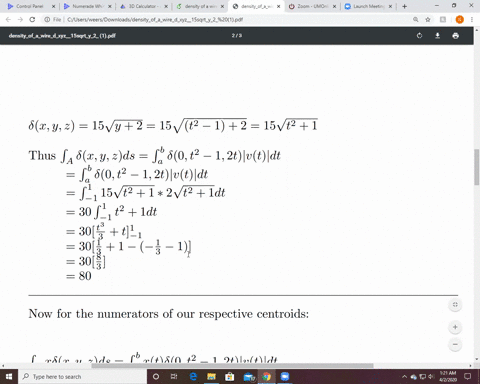🎉 The Study-to-Win Winning Ticket number has been announced! Go to your Tickets dashboard to see if you won! 🎉View Winning Ticket### Center of mass of a curved wire A wire of density…

21:35University of Central Arkansas
Problem 33

# Mass of a wire Find the mass of a wire that lies along the curve $\mathbf{r}(t)=\left(t^{2}-1\right) \mathbf{j}+2 t \mathbf{k}, 0 \leq t \leq 1,$ if the density is $\delta=(3 / 2) t$

## Discussion

You must be signed in to discuss.

## Video Transcript

Okay. What we want to do is we want to determine, um or fine the mass of a thin wire lying along this curve represented by r T is equal to the square root of two times T I plus the square root of two times T j plus foreplay four minus, I believe, um, four minus, um, t squared. Um, okay. And so, um and he's going to go from 0 to 1 inclusive in the first time. And so we know the mass is given by the integral over the curve of your, um, density function. It were integrating with respect to s. Okay. And the first time we're gonna do it is our density is given by three t. So what we needed to up here, what we need to do is actually to find d s. Um, and we know that d s is actually equal to, um the magnitude a v a t times t t. So what we need to do is find Viotti and V of tea is based off of the derivative of Artie. So we won't take the derivative of Artie, which is gonna give me the square root of two I plus the square root of two J minus two t k. And so the magnitude a V of tea is gonna be equal to the square root of the square root of two squared plus the square to two squared plus negative to tea. And we're gonna square that. And so we get, um, this equal to two times the square root of one plus t squared and so D s is two times the square root of one plus t squared TT So that's the first thing. That's the first thing we needed to do. Um, and now we need to set up our integral and so are integral is going to for the mass for the first time is from 0 to 1 of our density function, which is three t times two times a scrooge of one plus t squared DT. And so now we need to go ahead and integrate that. And so, um, the mass is equal to I'm gonna pull out the three from integral from 0 to 1, uh, to t times the square root a one plus t squared TT because I did that because I recognize I would have to do use up, and I might let you be one plus t squared. D'You is therefore to t t t. That's why I left that to tea or that two inside the integral. And so this now becomes the, um, three times the integral of you to the 1/2. Do you? And I'm gonna go ahead and change my upper lower limits. So when tea is zero us one when tea is one, you is two. And so this now becomes three times 2/3 you to the three halfs, and we're gonna evaluate it at two and at one. So this is too times two to the three halves, minus one, and this will become two times two times a squirter to minus one, which then I can distribute the two so four times it's quarter to minus two. So that's the first time that's the first mass. And now what we want to do is now, um, we want now the density function to be equal toe one. So how did that affect? So the math is equal to the integral from zero toe one of that density function, which is one times two times the square root of one plus t squared DT. And so this becomes two times integral from 0 to 1 of the screwed of one plus t squared T t. Okay, um and so now we've got a kind of integrate that integral. I mean, that square with a one plus t squared. And if you recollect, or you can look at a table that integral will become two. Um, because of the two out here, Ah, 1/2 times a t times this grow to one plus t squared, plus the natural log of the absolute value of tea plus the square root of one plus t squared. And, um, that is all multiplied by, um, the two in the 1/2. And so this now becomes, um, the two and the 1/2 um, multiply together to be a one. And so we have hope, and we're evaluating from okay, evaluating at one of and zero. So when I put in a one here, I get a square root of two, plus the natural log of one plus the square root of two, the minus. This whole term, when I do, zero becomes zero and this becomes the natural log of one, which is zero. And so the answer is the square root of two, plus the natural log of one plus it's quarter to.

#### You're viewing a similar answer. To request the exact answer, fill out the form below:

Our educator team will work on creating an answer for you in the next 6 hours.